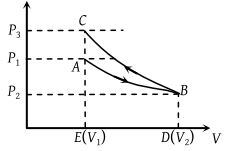# NEET Physics Thermodynamics Questions SolvedAn ideal gas expands isothermally from a volume V1 to V2 and then compressed to original volume V1 adiabatically. Initial pressure is P1 and final pressure is P3. The total work done is W. Then -

(1) ${P}_{3}>{P}_{1},\text{\hspace{0.17em}\hspace{0.17em}}W>0$

(2) ${P}_{3}<{P}_{1},\text{\hspace{0.17em}\hspace{0.17em}}W<0$

(3) ${P}_{3}>{P}_{1},\text{\hspace{0.17em}\hspace{0.17em}}W<0$

(4) ${P}_{3}={P}_{1},\text{\hspace{0.17em}\hspace{0.17em}}W=0$

Concept Videos :-

#19 | Work (W) done by the Gas: 1
#20 | Solved Example: 7
#21 | Work (W) done by the Gas: 2
#22 | Solved Example: 8
#23 | Solved Example: 9
#24 | Work (W) done by the Gas: 3
#25 | Graphical Intrepretation of Work done
#26 | Solved Example: 10
#27 | Solved Example: 11
#28 | Solved Example: 12

Concept Questions :-

Work done by gas

(3) From graph it is clear that P3 > P1.

Since area under adiabatic process (BCED) is greater than that of isothermal process (ABDE). Therefore net work done

$W={W}_{i}+\left(-{W}_{A}\right)$    $\left(\because \text{\hspace{0.17em}}{W}_{A}>{W}_{i}\right)$

W < 0Difficulty Level:

• 20%
• 33%
• 43%
• 6%
Crack NEET with Online Course - Free Trial (Offer Valid Till September 21, 2019)